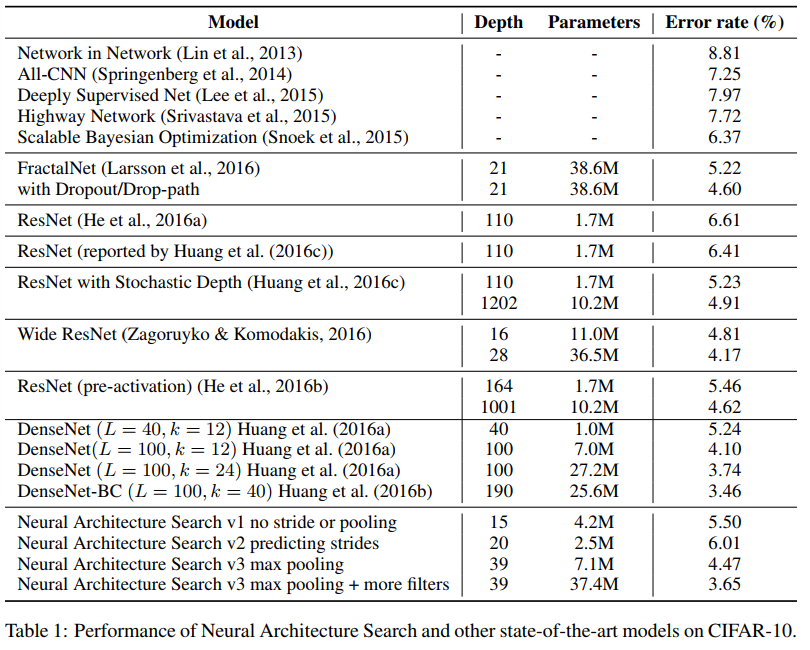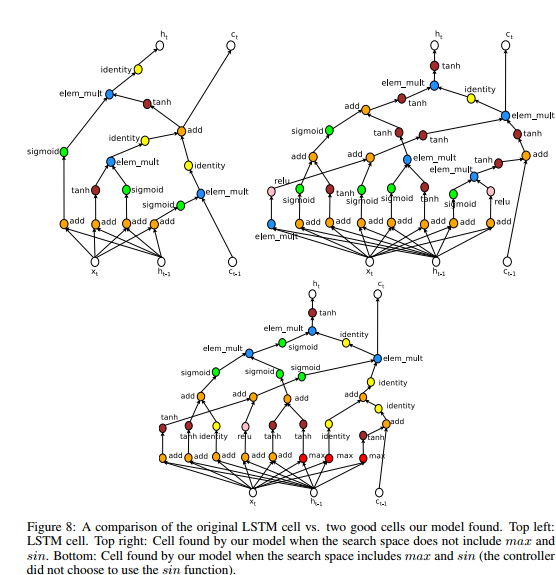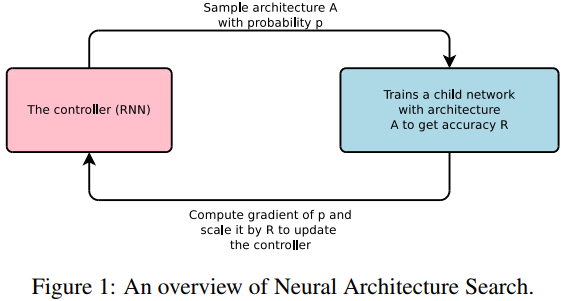The goal of this paper is to search for neural architectures. Since the architecture of a network can be seen as a sequence of elements, they predict a network A with an RNN controler (or policy network). Once A is generated, it is trained and its validation accuracy is used as reward to train the controler afterwards. More specifically, the controler is executed $$m$$ times, thus generating $$m$$ architectures $$A_i$$ to $$A_m$$. Then those $$m$$ networks are then trained and validated which results into $$m$$ validation accuracies (or rewards). The system then uses policy gradient to update the controler.

The actual policy gradient equation is as follows where $$T$$ is the number of hyperparameters the controller had to predict to design a neural network architecture and $$a_t$$ is the t-th element in the architecture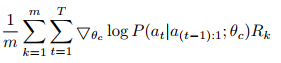Here is an exemple of a recurrent controler generating the structure of a simple CNN (the input is random noise)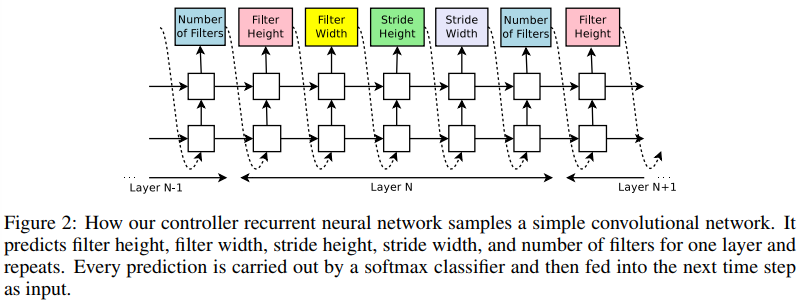The controler can also predict anchor points to get skip connections.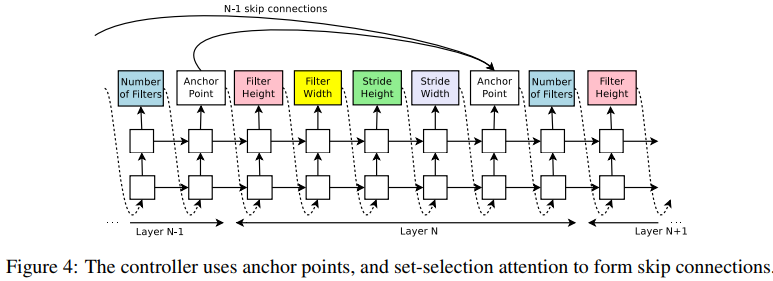They also proposed an RNN for predicting recurrent networks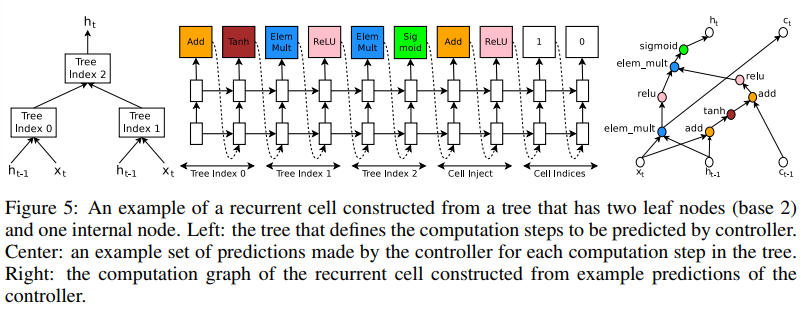## Results

The get state-of-the-art results on CIFAR-10 and outperform LSTM on Penn TreeBank